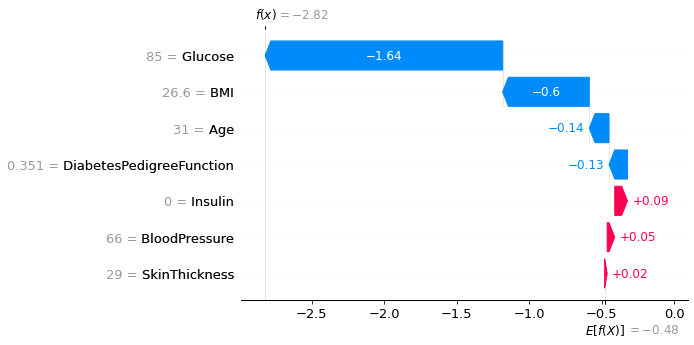# SHAP in Python

Interpretation of a Machine Learning model has been a longstanding issue. Many methods have been proposed over the last few years to use alternative approaches to the interpretation issue. I recently came across an article which uses SHAP (or Shapley Values), first introduced in 2017 in this paper.

SHAP or SHapley Additive exPlanations is a method to explain the results of running a machine learning model using game theory. The basic idea behind SHAP is fair allocation from cooperative game theory to allocate credit for a model’s output.

I will be using a sample data set to briefly explain how we can use this technique to our advantage.

Running the logistic regression code on the sample dataset.

`def get_stats():    X = data3[x_columns]    X_test = X.iloc[1:550,:]    Y_test = data3.iloc[1:550,k-1]    x = X_test    logit_model = sm.Logit(Y_test, sm.add_constant(X_test)).fit()    print(logit_model.summary())    get_stats()`

We will now apply a step-wise regression to find out the best model using some or all of the features of the above dataset.

We will now employ SHAP on our logistic regression model to figure out the most important features.

`import shapmasker = shap.maskers.Independent(data = X_test)model = LogisticRegression(random_state = 1)model.fit(X_train, Y_train)explainer = shap.LinearExplainer(model, masker=masker)shap_values = explainer(X_test)`

There are various ways to visualize the output of SHAP method.

`shap.plots.waterfall(shap_values)`Graph showing the extent to which each feature affect the output
`shap.plots.beeswarm(shap_values)`